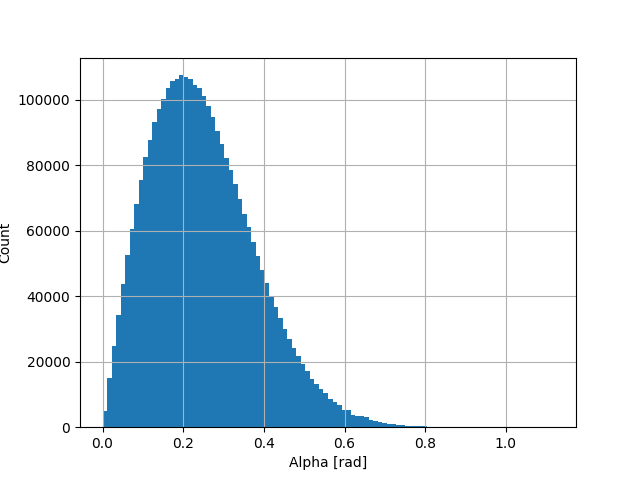# Optical surface

Hello friends.
What is the Definition of the SetSigmaAlpha() Method bellow? How does this function determine the roughness?
I mean, what are the maximum and minimum values of this function?

G4double sigma_alpha = 0.1;
OpSurface->SetType(dielectric_dielectric);
OpSurface->SetModel(unified);
OpSurface->SetFinish(groundbackpainted);
OpSurface->SetSigmaAlpha(sigma_alpha);

The angle alpha of a facet is calculated via:

This function code alpha to a random value taken from the
distribution p(alpha) = g(alpha; 0, sigma_alpha)*std::sin(alpha),
for alpha > 0 and alpha < 90, where g(alpha; 0, sigma_alpha)
is a gaussian distribution with mean 0 and standard deviation
sigma_alpha.